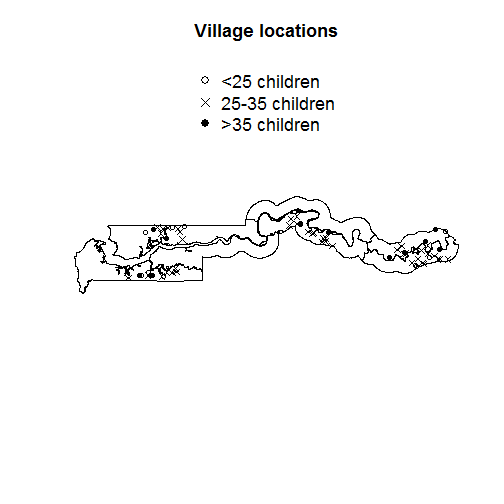# Model selection for the Gambia data

### Chapter 5.5: Model selection criteria

The gambia data in the geoR package includes data for 1332 children in the Gambia. The binary response $$Y_i$$ is the indictor that child $$i$$ tested positive for malaria. Child $$i$$ lives in village $$v_i\in \{1,…,65\}$$. We use five covariates in $$X_{ij}$$:

1. Age: age of the child, in days

2. Netuse: indicator variable denoting whether (1) or not (0) the child regularly sleeps under a bed-net

3. Treated: indicator variable denoting whether (1) or not (0) the bed-net is treated (coded 0 if netuse=0)

4. Green: satellite-derived measure of the greenness of vegetation in the immediate vicinity of the village (arbitrary units)

5. PCH: indicator variable denoting the presence (1) or absence (0) of a health center in the village

We use the random effects logistic regression model $\mbox{logit}[\mbox{Prob}(Y_i=1)] = \alpha + \sum_{j=1}^pX_{ij}\beta_j + \theta_{v_i},$ where $$\theta_v$$ is the random effect for village $$v$$. We compare three models for the village random effects via DIC and WAIC:

1. No random effects: $$\theta_v=0$$

2. Gaussian random effects: $$\theta_v\sim\mbox{Normal}(0,\tau^2)$$

3. Double-exponential random effects: $$\theta_v\sim\mbox{DE}(0,\tau^2)$$

## Load the data

library(geoR)

Y <- gambia[,3]
X <- scale(gambia[,4:8])
s <- gambia[,1:2]
n <- length(Y)
p <- ncol(X)

# Compute the village ID

S <- unique(s) # Lat/long of the villages
m <- nrow(S)
village <- rep(0,n)
members <- rep(0,m)
for(j in 1:m){
d             <- (s[,1]-S[j,1])^2 + (s[,2]-S[j,2])^2
village[d==0] <- j
members[j]    <- sum(d==0)
}

size <- ifelse(members<25,1,2)
size <- ifelse(members>35,3,size)
table(size)

## size
##  1  2  3
## 11 42 12

pch  <- c(1,4,19)

plot(gambia.borders, type="l",
asp=1,axes=F,cex.main=1.5,
xlab="",ylab="",main="Village locations")
points(S[,1],S[,2],pch=pch[size])
legend("top",c("<25 children","25-35 children",">35 children"),pch=pch,cex=1.5,bty="n")## Prep for JAGS

   library(rjags)
burn   <- 1000
iters  <- 5000
chains <- 2


## Model 1: No random effects

   mod <- textConnection("model{
for(i in 1:n){
Y[i]          ~ dbern(pi[i])
logit(pi[i]) <- beta        + X[i,1]*beta + X[i,2]*beta +
X[i,3]*beta + X[i,4]*beta + X[i,5]*beta
like[i]      <- dbin(Y[i],pi[i],1) # For WAIC computation
}
for(j in 1:6){beta[j] ~ dnorm(0,0.01)}
}")

data   <- list(Y=Y,X=X,n=n)
model  <- jags.model(mod,data = data, n.chains=chains,quiet=TRUE)
update(model, burn, progress.bar="none")
samps  <- coda.samples(model, variable.names=c("like"),
n.iter=iters, n.thin = 5, progress.bar="none")

# Compute DIC
DIC    <- dic.samples(model,n.iter=iters,n.thin = 5, progress.bar="none")

# Compute WAIC
like   <- rbind(samps[],samps[]) # Combine samples from the two chains
fbar   <- colMeans(like)
Pw     <- sum(apply(log(like),2,var))
WAIC   <- -2*sum(log(fbar))+2*Pw

DIC

## Mean deviance:  2520
## penalty 6.076
## Penalized deviance: 2526

   WAIC;Pw

##  2525.59

##  6.029153


## Model 2: Gaussian random effects

   mod <- textConnection("model{
for(i in 1:n){
Y[i]          ~ dbern(pi[i])
logit(pi[i]) <- beta        + X[i,1]*beta + X[i,2]*beta +
X[i,3]*beta + X[i,4]*beta + X[i,5]*beta +
theta[village[i]]
like[i]      <- dbin(Y[i],pi[i],1) # For WAIC computation
}
for(j in 1:6){beta[j] ~ dnorm(0,0.01)}
for(j in 1:65){theta[j] ~ dnorm(0,tau)}
tau   ~ dgamma(0.1,0.1)
}")

data   <- list(Y=Y,X=X,n=n,village=village)
model  <- jags.model(mod,data = data, n.chains=chains,quiet=TRUE)
update(model, burn, progress.bar="none")
samps  <- coda.samples(model, variable.names=c("like"),
n.iter=iters, n.thin = 5,progress.bar="none")

# Compute DIC
DIC    <- dic.samples(model,n.iter=iters,n.thin = 5,progress.bar="none")

# Compute WAIC
like   <- rbind(samps[],samps[])
fbar   <- colMeans(like)
Pw     <- sum(apply(log(like),2,var))
WAIC   <- -2*sum(log(fbar))+2*Pw

DIC

## Mean deviance:  2278
## penalty 54.85
## Penalized deviance: 2333

   WAIC;Pw

##  2333.474

##  53.43145


## Model 3: Double-exponential random effects

   mod <- textConnection("model{
for(i in 1:n){
Y[i]          ~ dbern(pi[i])
logit(pi[i]) <- beta        + X[i,1]*beta + X[i,2]*beta +
X[i,3]*beta + X[i,4]*beta + X[i,5]*beta +
theta[village[i]]
like[i]      <- dbin(Y[i],pi[i],1) # For WAIC computation
}
for(j in 1:6){beta[j] ~ dnorm(0,0.01)}
for(j in 1:65){theta[j] ~ ddexp(0,tau)}
tau   ~ dgamma(0.1,0.1)
}")

data   <- list(Y=Y,X=X,n=n,village=village)
model  <- jags.model(mod,data = data, n.chains=chains,quiet=TRUE)
update(model, burn, progress.bar="none")
samps  <- coda.samples(model, variable.names=c("like"),
n.iter=iters, n.thin = 5,progress.bar="none")

# Compute DIC
DIC    <- dic.samples(model,n.iter=iters,n.thin = 5,progress.bar="none")

# Compute WAIC
like   <- rbind(samps[],samps[])
fbar   <- colMeans(like)
Pw     <- sum(apply(log(like),2,var))
WAIC   <- -2*sum(log(fbar))+2*Pw

DIC

## Mean deviance:  2276
## penalty 56.91
## Penalized deviance: 2333

   WAIC;Pw

##  2333.153

##  54.29588


Summary: Both $$WAIC$$ and $$DIC$$ show strong support for including village random effects but cannot distinguish between Gaussian and double-exponential random effect distributions.